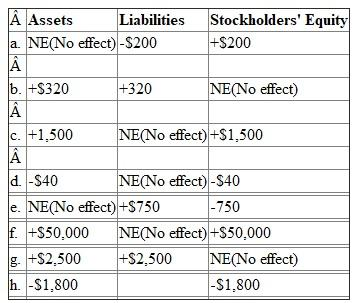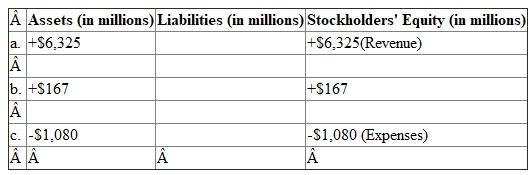# Quiz 5: Fraud, Internal Control, and Cash

Effect of transactions on Accounting equation The following table shows the effect of the given transactions on the Accounting equation.a. Unused gift certificates are liabilities of the company. When they are used by the customers, the journal entry will be recorded by debiting Gift certificates (liability) account and crediting Revenue account. Revenues will increase shareholders' equity. b. Equipment acquired on account will increase Equipment account(asset account) and increase Accounts payable account(liability) c. Revenues earned on account will increase revenue account(shareholders' equity) and Accounts receivable account(asset) d. Advertising expense incurred is recorded by debiting expenses account which will reduce shareholders' equity and by crediting cash account which will reduce assets e. Accrued utility bills are liabilities. Therefore liabilities are increased and shareholders' equity is decreased due to the expenses which are incurred f. Issue of shares will increase the shareholders' account and cash (asset) account g. Cash received on notes payable will increase cash account and notes payable (liability) accounts h. Depreciation expense recorded will reduce net profit, hence will reduce shareholders' expense. It will be deducted from the asset which is depreciated , reducing the asset account

There is no answer for this question

Effect of transactions on Accounting equation The following table shows the effect of the given transactions on the Accounting equation.a. Revenues on account increases revenues (share holders' equity) and bills receivable (asset) account b. Issue of stock increases capital (share holders' equity) and cash (asset) account c. Expenses paid in cash reduce cash (asset) and shareholders' equity. The accounting equation after recording the transactions will be:There is no answer for this question

There is no answer for this question

There is no answer for this question

There is no answer for this question

There is no answer for this question

There is no answer for this question

There is no answer for this question